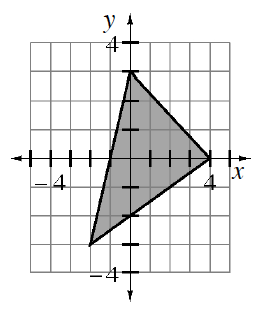### Home > CCA2 > Chapter 4 > Lesson 4.2.4 > Problem4-100

4-100.

Write the three inequalities that form the triangle shown at right.

Start by finding the equations that correspond to the line segments that form the sides of the triangle.

Determine whether the triangle lies above (greater than) or below (less than) the line.

Left side: Since the $y$-intercept is $(0,3)$ and the slope is $3$, the equation of the line is $y=3x+3$.

The shaded region lies below the line. Which symbol should you use? You can test to be sure you are correct by substituting $(0,0)$ into your inequality to be sure it is true.

$y\le3x+3$

Now find the other two inequalities.